Documentation

## Array Modeling and Analysis

This example shows how to construct, visualize, and analyze an antenna array from the Antenna Toolbox.

### Create Antenna Array Using Antenna Elements

Create a default rectangular antenna array using the `rectangularArray` element in the array library. By default, the array uses the dipole as an antenna element.

```ra = rectangularArray ```
```ra = rectangularArray with properties: Element: [1x1 dipole] Size: [2 2] RowSpacing: 2 ColumnSpacing: 2 Lattice: 'Rectangular' AmplitudeTaper: 1 PhaseShift: 0 Tilt: 0 TiltAxis: [1 0 0] ```

### Visualize Layout of Array

Use the `layout` function to plot the position of array elements in the x-y plane. By default, the rectangular array is a 4-element dipole array in a 2x2 rectangular lattice.

```layout(ra) ```### Visualize Geometry of Array

Use the `show` function to view the structure of the rectangular antenna array.

```show(ra) ```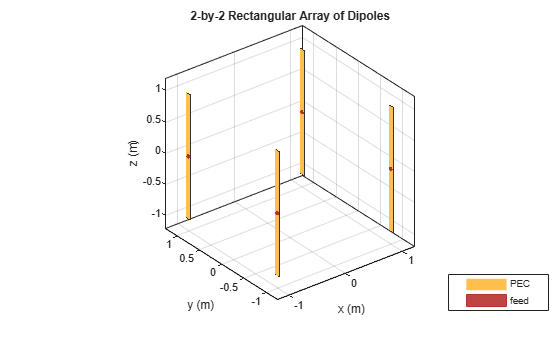### Plot Radiation Pattern of Array

Use the `pattern` function to plot the radiation pattern of the rectangular array. The radiation pattern is the spatial distribution of the power of an array. The pattern displays the directivity or gain of the array. By default, the pattern function plots the directivity of the array.

```pattern(ra,70e6) ```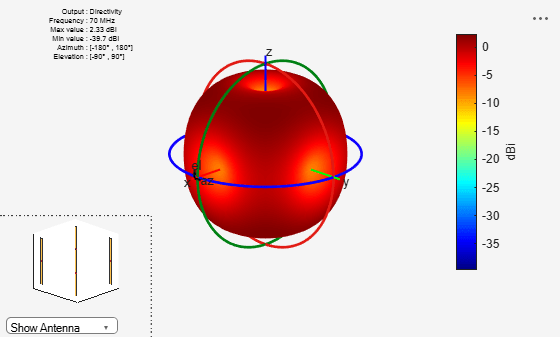### Plot Azimuth and Elevation Pattern of Array

Use `patternAzimuth` and `patternElevation` functions to plot the azimuth and elevation pattern of the rectangular array. These two patterns are the 2D radiation pattern of the array at a specified frequency.

```patternAzimuth(ra,70e6) figure patternElevation(ra,70e6) ```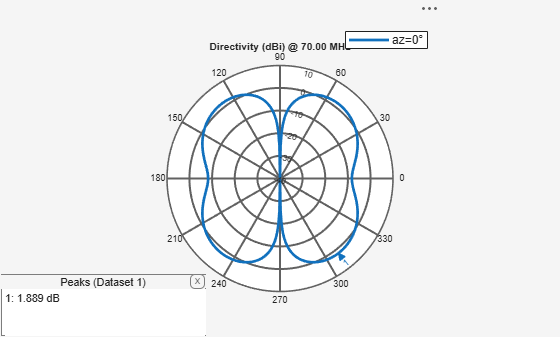### Calculate the Directivity of Array

Directivity is the ability of an array to radiate power in a particular direction. It can be defined as the ratio of the maximum radiation intensity in the desired direction to the average radiation intensity in all other directions. Use the `pattern` function to calculate the directivity of the rectangular array.

```[Directivity] = pattern(ra,70e6,0,90) ```
```Directivity = -40.1001 ```

### Calculate EH Fields of Array

Use the `EHfields` function to calculate the EH fields of the rectangular array. EH fields are the x, y, and z components of the electric and magnetic fields of an array. These components are measured at a specific frequency and at specified points in space.

```[E,H] = EHfields(ra,70e6,[0;0;1]) ```
```E = -0.0000 - 0.0000i -0.0002 + 0.0002i -1.3304 - 0.0758i H = 1.0e-05 * -0.1274 - 0.1541i -0.0000 - 0.0000i 0.0000 + 0.0000i ```

### Plot Different Polarizations of Array

Use the Polarization name-value pair in the pattern function to plot the different polarization patterns of the rectangular array. Polarization is the orientation of the electric field, or E-field, of an array. Polarization is classified as elliptical, linear, or circular. This example shows the left-hand circularly polarized (LHCP) radiation pattern of the rectangular array.

```pattern(ra,70e6,'Polarization','LHCP') ```### Calculate Beamwidth of Array

Use the `beamwidth` function to calculate the beamwidth of the rectangular array. The beamwidth of an array is the angular measure of the array pattern coverage. The beamwidth angle is measured in the plane that contains the direction of main lobe of the array.

```[bw,angles] = beamwidth(ra,70e6,0,1:1:360) ```
```bw = 44.0000 44.0000 angles = 288 332 208 252 ```

### Calculate Scan Impedance of Array

Use the `impedance` function to calculate and plot the input impedance of rectangular array. Active impedance, or scan impedance, is the input impedance of each antenna element in an array, when all elements are excited.

```impedance(ra,60e6:1e6:70e6) ```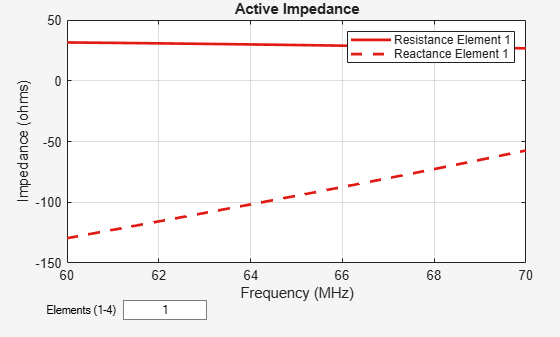You can also view the impedance of all four elements by changing the number of elements on the plot from 1 to 1:4. See figure.### Calculate Reflection Coefficient of Array

Use the `sparameters` function to calculate the S11 value of the rectangular array. S11 value gives the reflection coefficient of the array.

```S = sparameters(ra,60e6:1e6:70e6,72) rfplot(S) ```
```S = sparameters: S-parameters object NumPorts: 4 Frequencies: [11x1 double] Parameters: [4x4x11 double] Impedance: 72 rfparam(obj,i,j) returns S-parameter Sij ```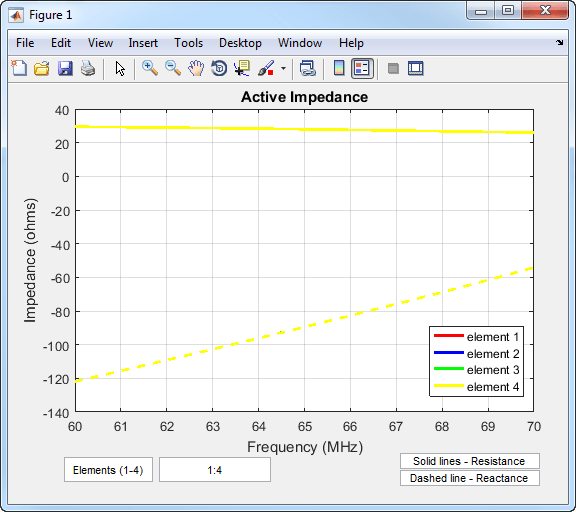### Calculate Return Loss of Array

Use the `returnLoss` function to calculate and plot the return loss of the rectangular array.

```returnLoss(ra,60e6:1e6:70e6,72) ```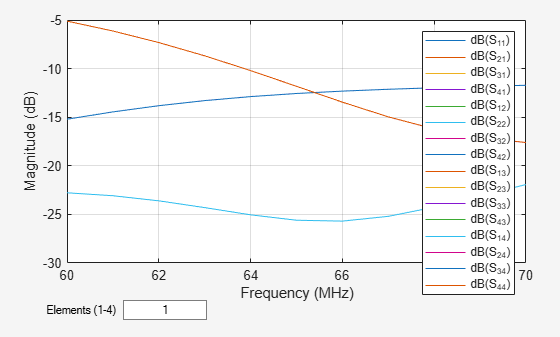You can also view the return loss of all four elements by changing the number of elements on the plot from 1 to 1:4. See figure.### Calculate Charge and Current Distribution Of Array

Use the `charge` and `current` functions to calculate the charge and current distribution on the rectangular array surface.

```charge(ra,70e6) figure current(ra,70e6) ```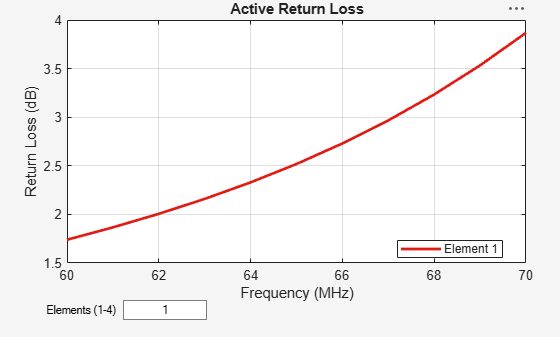### Calculate Correlation Coefficient of Array

Use the `correlation` to calculate the correlation coefficient of the rectangular array. The correlation coefficient is the relationship between the incoming signals at the antenna ports in an array.

```correlation(ra,60e6:1e6:70e6,1,2) ```### Change Size of Array and Visualize Layout

Use the 'Size' property of the rectangular array to change it to a 16-element dipole array.

```ra.Size = [4 4]; show(ra) ```### Change Array Elements Spacing To Nonuniform

Use the 'RowSpacing' and 'ColumnSpacing' properties of rectangular array to change the spacing between the antenna elements.

```ra.RowSpacing = [ 1.1 2 1.2]; ra.ColumnSpacing =[0.5 1.4 2] show(ra) ```
```ra = rectangularArray with properties: Element: [1x1 dipole] Size: [4 4] RowSpacing: [1.1000 2 1.2000] ColumnSpacing: [0.5000 1.4000 2] Lattice: 'Rectangular' AmplitudeTaper: 1 PhaseShift: 0 Tilt: 0 TiltAxis: [1 0 0] ```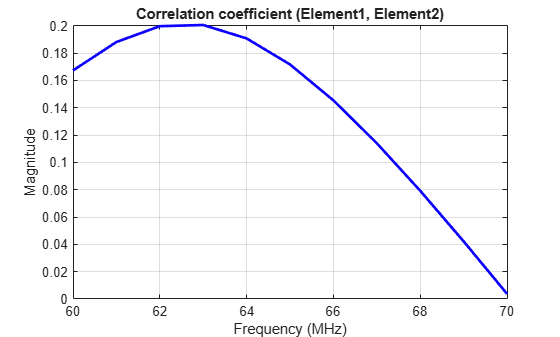### References

 Balanis, C.A. "Antenna Theory. Analysis and Design", p. 514, Wiley, New York, 3rd Edition, 2005.